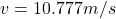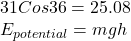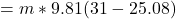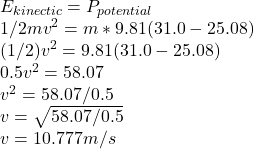Question

Tarzan swings on a 31.0 m long vine initially inclined at an angle of 36.0◦ with the vertical. The acceleration of gravity if 9.81 m/s 2 . What is his speed at the bottom of the swing if he a) starts from rest? Answer in units of m/s

1.Explanation:

Tarzan swing can be thought of as change in potential energy by going from higher location We solve for height of beginning of the swing by using simple cosine equation:

SoΔE=mg(h₂-h₁)The potential energy of Tarzan initial position is converted into kinetic energy of his swing.By using kinetic equation